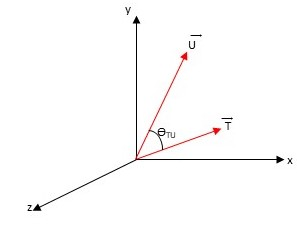# Problem: The figure shows two vectors T_vec and U_vec separated by an angle theta_TU. (Figure 1) You are given that vec{T} = (3,1,0), vec{U} = (2,4,0), and vec{T} × vec{U} = vec{V}. Express V_vec as an ordered triplet of values, separated by commas.

###### FREE Expert Solution

99% (473 ratings)###### Problem Details

The figure shows two vectors T_vec and U_vec separated by an angle theta_TU. (Figure 1) You are given that vec{T} = (3,1,0), vec{U} = (2,4,0), and vec{T} × vec{U} = vec{V}.

Express V_vec as an ordered triplet of values, separated by commas.